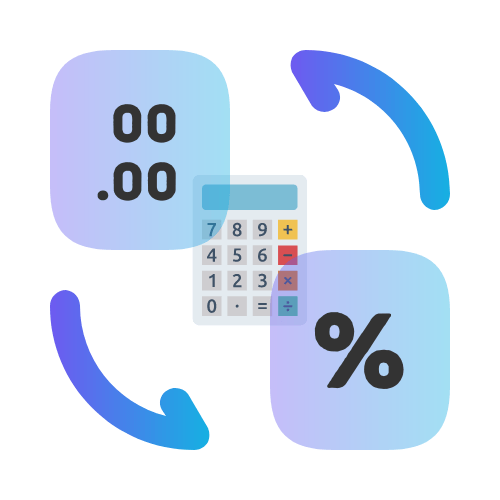# Decimal to Percent Calculator

## Convert decimals to percentages with this calculator

• Created by John Harris
• Reviewed by Ramesh Agarwal
Enter a number.

The result is:

## Why Convert Decimal to Percentages?

Converting decimal numbers to percentages can be a good tool for creating a better user experience. It can help your users to make more informed choices when they are reading your website, or when they are looking at your products. Showing numbers in percentage form is also a great way to communicate how much you are offering in a sale.

## Can percentages be decimals?

It is a very common practice to show numbers as a percentage - it's a quick and easy way to show relative measurements. Many businesses want to display their numbers in percentages, as it is useful to show useful metrics like sales growth or performance measurements within a sales team.

A percentage is a number expressed as a fraction of one hundred, and a decimal number is a number expressed as a fraction of one. So, if a decimal is a fraction of one, then what happens when you multiply a decimal number by 100? The answer is that you get a percentage.

## When to convert a decimal number to percentage?

When converting decimal into percentage, you have to consider what you are trying to achieve. You can convert a decimal number to a percentage to show the actual size of the number in relation to the whole. In other words, you will see what percentage the number is of the total. For example, 0.2 is shown as 20%.

Converting decimal numbers to percentage (or percent to decimal) can be useful when you are trying to compare two numbers. For example, let's say you are trying to compare the sales of two products and you want to see which one is more popular. The easiest way to do this is to convert the sales to percentage and then compare the numbers.

## Should I display numbers as decimal or percent?

When it comes to displaying numbers, it can sometimes be confusing which number format to use. Sometimes even experienced marketers will use the wrong number format to display the number and that can cost a lot of money. But the question is: "which number format should I use"? The answer is both, for different scenarios.

You should use the percentage number format for the amount of a purchase or the amount of a sale and you should use the decimal number format for percentages that are used to show a ratio or a fraction.

## How many decimal places are in a percentage?

When it comes to showing numbers in percentages, there are a couple of ways to do it. One way is to simply use the number and the percent sign, but most of the time you will see percentages written as decimals and then an asterisk to show that it is a percentage. However, many people wonder how many decimal places to show in a percentage. Well, it depends.

Normally percentages are rounded to the nearest whole number, as it is easier for us to understand at a glance. However, there are times when you want to be more precise with calculations. An example of this is when showing financial reports, in such cases it may be more appropriate to use 2 decimal places.

## Should I use decimals rather than percentages?

The decimal system is the common way to write numbers, but is it always the best way? There are situations when it can be a better idea to use percentage form, or as it's also know as, percentage notation. As an example, if you want to know how much your sales have grown you might like to show this as a percentage.

If you are showing the amount of something in a limited quantity such as a number of customers, then it might be best to use percentage notation. Percentage notation can also be useful if you want to show the benefit of a discount or the amount of a discount.

One of the easiest ways to convert between decimals and percentages is to use a tool like the decimal to percentage calculator. This calculator makes it easy as you don't need to do any custom calculations. Just enter the decimal and click the button.

## What is the decimal to percent formula?

You can easily convert a decimal to percentage by multiplying it by 100. For example, you can convert 0.25 to percentage by multiplying it by 100: 0.25 * 100 = 25%. The calculation is quite simple but for many of us who don't use this kind of Math on a daily basis, it is normal to forget the formula. This is why it can be helpful to use a decimal to percent calculator to convert a percentage to a decimal instantly.

## FAQS

To convert a decimal to a percent, you multiply the decimal by 100. This will give you the equivalent percentage value.

The decimal form of 75% is 0.75. To convert a percentage to a decimal, divide the percentage value by 100.

Sure! For example, to convert the decimal 0.6 to a percent, you multiply it by 100 to get 60%.

To express a fraction as a percentage, first convert the fraction to a decimal and then multiply it by 100 to get the percentage value.

The percentage equivalent of 0.25 is 25%. This is because 0.25 multiplied by 100 gives 25.

Yes, you can also move the decimal point two places to the right. For example, 0.8 becomes 80% when converted to a percent.